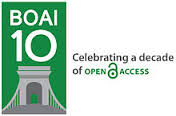### INFLUENCE OF ICT-LEARNED MATHEMATICS LEARNING MEDIA IN STUDENT LEARNING CHILDREN

Sulaiman Sulaiman

#### Abstract

ABSTRACT

This study aims to determine: 1) whether there is influence of mathematics learning using ICT-based mathematics learning media with lessons applied without using ICT-based mathematics learning media on statistical materials, 2) Which is higher mean mathematics learning outcomes using media learning mathematics based ICT with applied learning without using ICT-based mathematics learning media on statistical materials. This research was conducted in SMA N 1 Adiluwih with the reason the teacher in the school is still limited in utilizing ICT in learning mathematics.

This research is a quasi-experimental research consisting of two variables that is independent variable in the form of direct learning using learning media in the form of Minitab and dependent variable that is average of mathematics learning result of student of class XI odd semester SMA N 1 Adiluwih academic year 2016-2017. To obtain student learning result data of statistical subject matter used test. To determine the validity of a test, the researcher uses the validity of the content and the validity of the item. Test reliability is done to determine the level of sharpness or accuracy of tests used with the alpha formula. Population in this research is all student of class XI odd semester SMA N 1 Adiluwih academic year 2016-2017 amount 100 student by taking sample as much as two class. In research sampling, the researcher uses cluster random sampling technique, in relation to the study population in the form of groups consisting of 3 classes and each class has the same characteristics, the sample determination is done by lottery. Data analysis technique Data analysis is intended to perform hypothesis testing and answer the proposed problem formulation, hypothesis testing in this study using t-test statistic.

Based on the result of the research, it can be concluded that in the material of odd semester statistics SMA N 1 Adiluwih academic year 2016/2017: 1) the average of mathematics learning result by using ICT-based mathematics learning media is higher than those that do not use ICT based mathematics learning media that is 72,36 and 66,59. 2) learning mathematics by using ICT-based mathematics learning media influence on student's mathematics learning outcomes on the subject of statistics.

Keywords: mathematics learning media, minitab, statistics

### Refbacks

• There are currently no refbacks.-----------------------------------------------------------------------------------------------------------------------------

Indexed by :ĀĀ

ĀICSTIEM: International Conference on Sosial Technological, Innovation, Economics and Management, Penerbit Fakultas Ilmu Sosial dan Ilmu PolitikĀ(http://www.jurnal.saburai.ac.id/index.php/ICSTIEM/index).